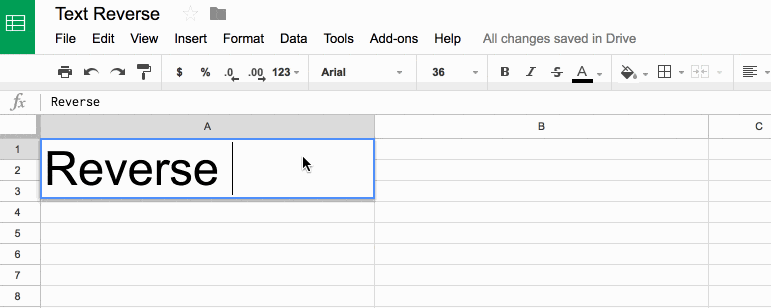## 10 Tips To Build A Google Sheets Budget Template

It probably won’t surprise you to hear that I use a Google Sheets budget template to track my finances, both incomings and outgoing, at home and for my business.

The dashboards available through online banking sites are pretty rudimentary. They don’t give much insight into what’s happening with my finances, particularly over longer time frames.

I like using Google Sheets, as opposed to another third party service like Mint, because it’s fully customizable. It’s easy to use and I can share any spending or budget templates easily with my wife.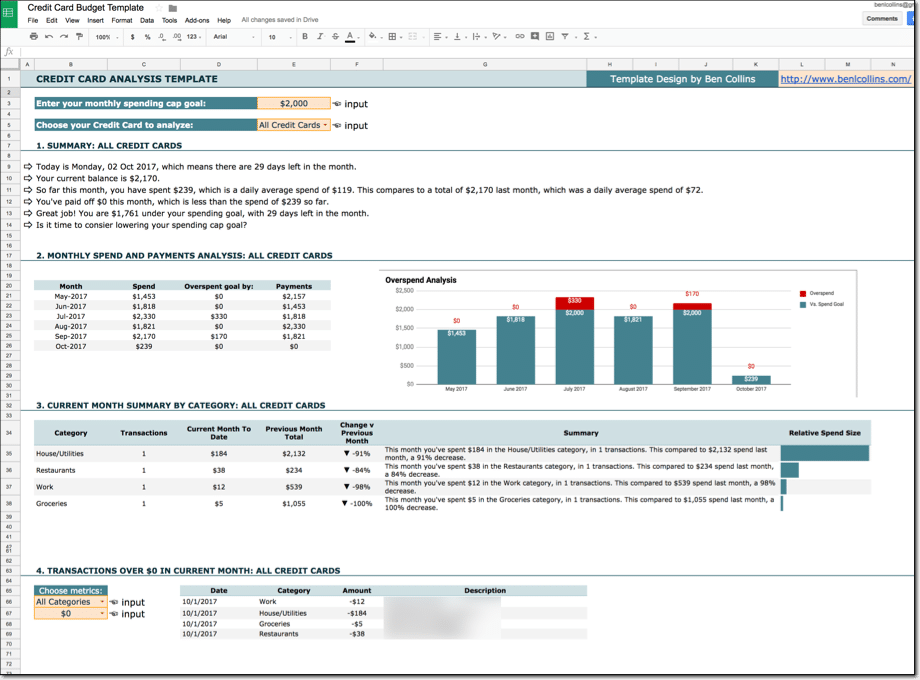I’m not a financial expert, so I won’t be dispensing any financial advice here. I won’t opine on what you should or shouldn’t show in your spending and budget templates in this post, nor will I talk about what your financial goals should be or how to get there.

What I will do in this post however, is show you some useful tips in Google Sheets that you can use for building your own budget templates. Techniques to make them more insightful and more helpful for reaching your goals.

## Filtering With Dates In The QUERY Function

If you’ve ever tried to filter on a date column in the Query function in Google Sheets, then you know how tricky it can be.

In a nutshell, the problem occurs because dates in Google Sheets are actually stored as serial numbers, but the Query function requires a date as a string literal in the format yyyy-mm-dd, otherwise it can’t perform the comparison filter.

This post explores this issue in more detail and shows you how to filter with dates correctly in your Query formulas.

## The problem

You might for example try the following syntax:

`=QUERY(Data!\$A\$1:\$H\$136,"select C, B where B > '1/1/2000'",1)`

Unfortunately, the output of such a query is blank: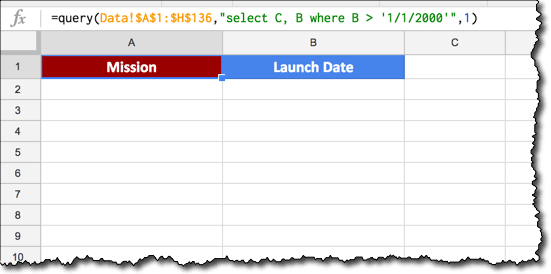If instead we remove the single quotes from around the date and try again, we get a #VALUE! error because the Query formula can’t perform the comparison: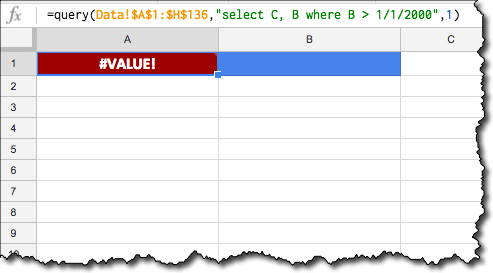Alas, what are we to do!

Neither of these “standard” formats work, because the dates are not in the correct format for the Query function.

## Correct syntax for dates in the Query function

Per the Query Language documentation, we need to include the `date` keyword and ensure that the date is in the format `yyyy-mm-dd` to use a date as a filter in the WHERE clause of our Query function.

Putting aside the Query function for a moment, let’s consider that `"select..."` string.

The new syntax we want will look like this:

`date_column > date '2000-01-01'`

Our challenge is to create a text formula to create this syntax for us, inside our query function.

Dealing with the text function first, starting with our required date of 1/1/2000 and working outwards:

First, we convert it to a serial number format with the `DATEVALUE()` wrapper:

`=DATEVALUE("1/1/2000")`

The output of this formula is a number:

`36526`

Then the `TEXT()` function converts it to the required format for the Query formula by specifying a format of `"yyyy-mm-dd"`:

`=TEXT(DATEVALUE("1/1/2000"),"yyyy-mm-dd")`

The output of this formula is a date in the desired format:

`2000-01-01`

Next we add single quotes around the new date format, with the `"'"` syntax. Finally, we insert the word `date` into the query string, to give:

`="select C, B where B > date '"&TEXT(DATEVALUE("1/1/2000"),"yyyy-mm-dd")&"'"`

which gives or desired output:

`select C, B where B > date '2000-01-01'`

That’s the syntax challenge done!

We can now plop that string into the middle argument of our Query function as per usual, and it’ll do the trick for us.

In this case, I was using a table of Space Shuttle mission data from Wikipedia, which contains a column of launch dates.

I used the `IMPORTHTML()` function to import that table into my Google Sheet, into a tab called Data in the range `A1:H136`. There’s a link to this dataset and worksheet at the end of the post.

This Query formula returns all of the Space Shuttle missions after 1 January 2000:

`=QUERY(Data!\$A\$1:\$H\$136,"select C, B where B > date '"&TEXT(DATEVALUE("1/1/2000"),"yyyy-mm-dd")&"'",1)`

The output of our formula is now returning the correct, filtered data: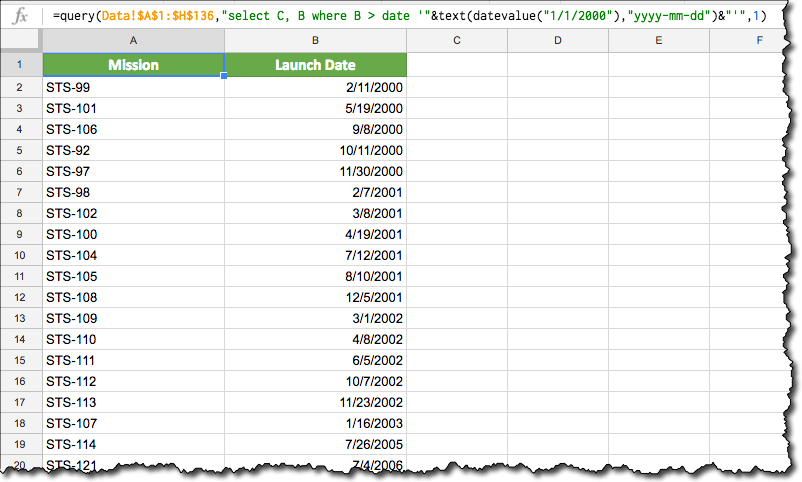## Referencing a date in a cell

The formula is actually simpler in this case, as we don’t need the DATEVALUE function. Assuming we have a date in cell A1 that we want to use in our filter, then the formula becomes:

`=QUERY(Data!\$A\$1:\$H\$136,"select C, B where B > date '"&TEXT(A1,"yyyy-mm-dd")&"'",1)`

## Example showing filter between two dates

Again, it’s relatively simple to extend our formula by adding a second date clause after the `AND` keyword:

`=QUERY(Data!\$A\$1:\$H\$136,"select C, B where B > date '"&TEXT(A1,"yyyy-mm-dd")&"' and B <= date '"&TEXT(B1,"yyyy-mm-dd")&"'",1)`

## Using today’s date as a filter

Substitute the TODAY() function into our formula:

`=QUERY(Data!\$A\$1:\$H\$136,"select C, B where B > date '"&TEXT(TODAY(),"yyyy-mm-dd")&"'",1)`

## How to add a total row to a Query Function table in Google Sheets

This article looks at how to add a total row to tables generated using the Query function in Google Sheets. It’s an interesting use case for array formulas, using the `{...}` notation, rather than the `ArrayFormula` notation.

So what the heck does this all mean?

It means we’re going to see how to add a total row like this:

using an array formula of this form:

`= { QUERY ; { "TOTAL" , SUM(range) } }`

## Multi-colored line charts in Google Sheets

In this article, you’ll see how to create a multi-colored line chart in Google Sheets, for example when the line is increasing it’s colored green, when it’s decreasing it’s colored red, as shown in this image: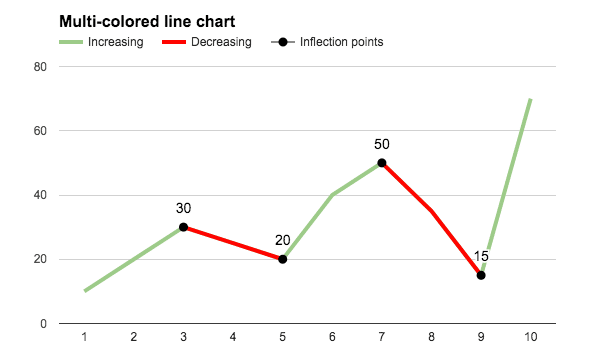The embedded charts tool in Google Sheets is pretty basic, so we can only achieve this with a formula workaround.

## How do I create a multi-colored line chart in Google Sheets?

### Basic Example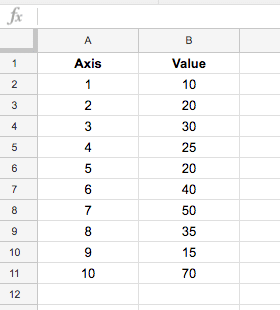which, when charted, looks like this: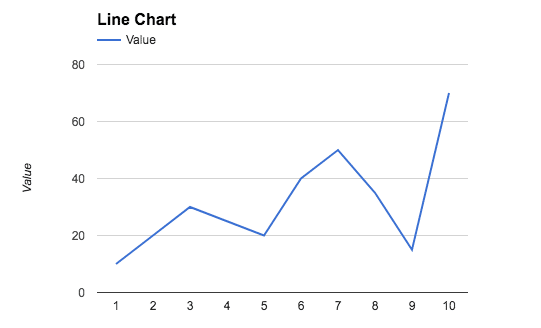To create the colored version seen at the top of this post, we need to add helper columns to the dataset, one to create a dataset of decreasing values, and an optional column to mark the inflection points (where the line changes from going up to going down, or vice versa).

The finished dataset looks like this: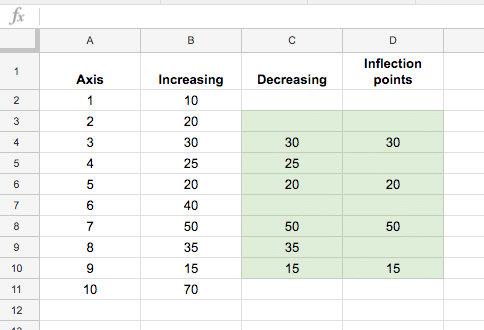The green highlighted cells contain formulas to calculate the decreasing data and the inflection points (see below). The first and last lines in column C and D (cells C2, D2, C11, D11 in this case) are left blank.

The IF function in column C, starting in cell C3 down to C10 is:

`=IF(OR(B3>B4,B3<B2),B3,"")`

The formula in column D, for identifying inflection points, starting in cell D3 down to D10, is:

`=IF(OR(B3=MAX(B2:B4),B3=MIN(B2:B4)),B3,"")`

With this data table setup, highlight the whole table (use Ctrl + A, or Cmd + A on a Mac, to do this quickly) and `Insert > Chart...`: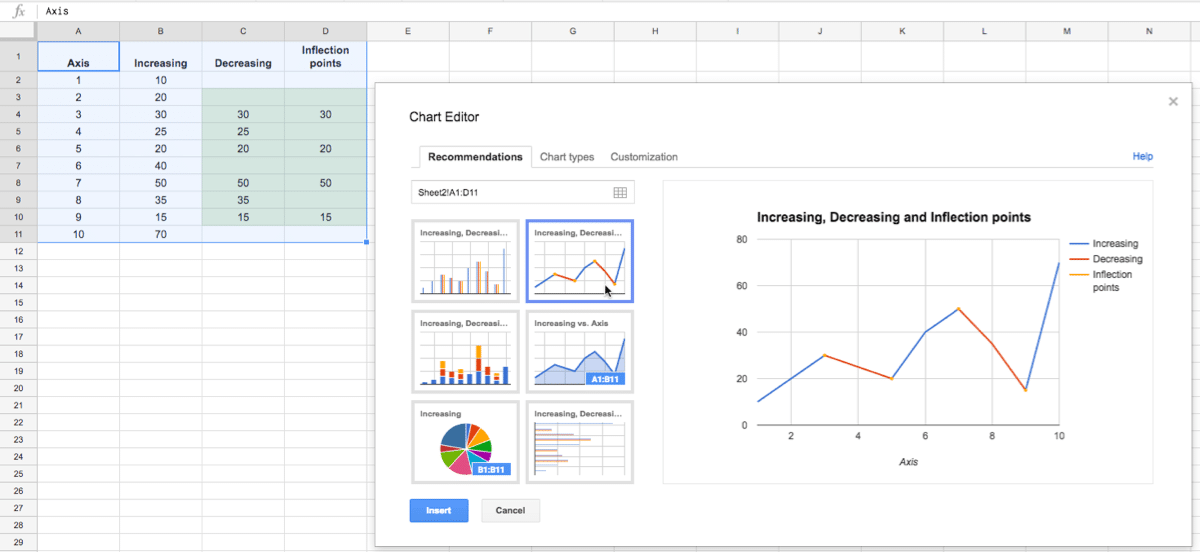Then simply format to the style you want, such as coloring the Increasing Series in green and the Decreasing Series in red:### Problem with this basic interpretation of this chart

This method has a drawback though, if you have adjacent inflection points, i.e decreasing – increasing – decreasing, then it tricks the chart so it colors the whole section decreasing, as shown in this image: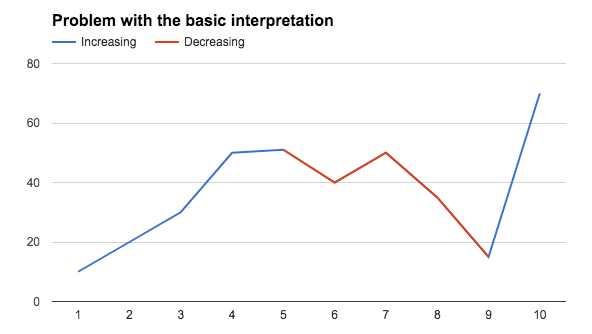### The fix

If you encounter this issue of adjacent inflection points, then you’ll need to create additional decreasing series to separate them, like this example dataset: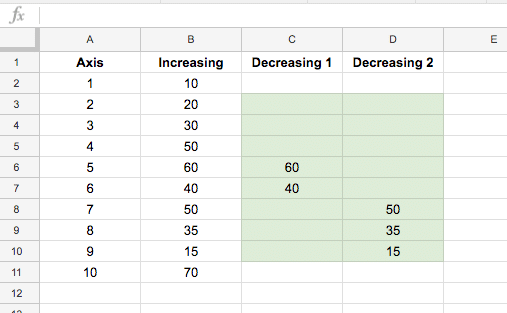The final chart will then look like this: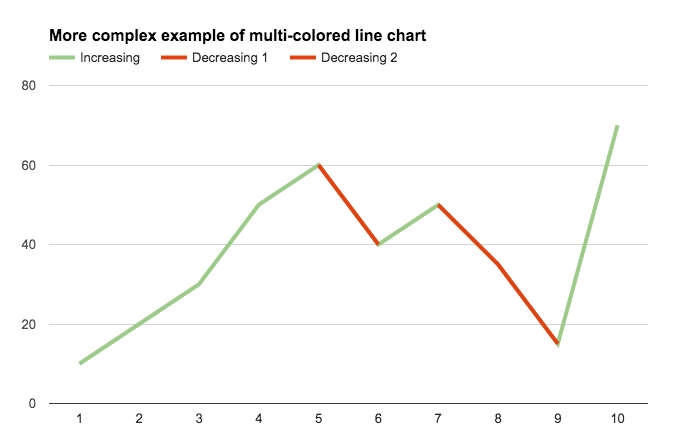Again we need to split the inflection point data into two columns so there are no adjacent inflection points in these series. The dataset now looks like: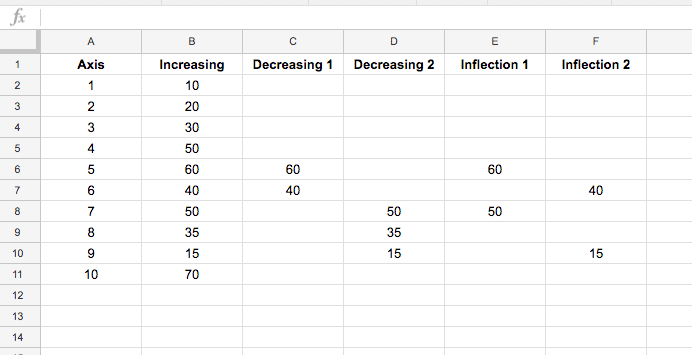and the final chart: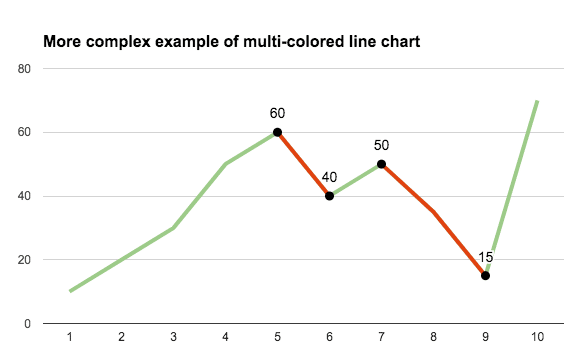### Even more customization options

Select the Combo chart instead of the straightforward line chart and change the increasing series to a line and the decreasing series to area charts: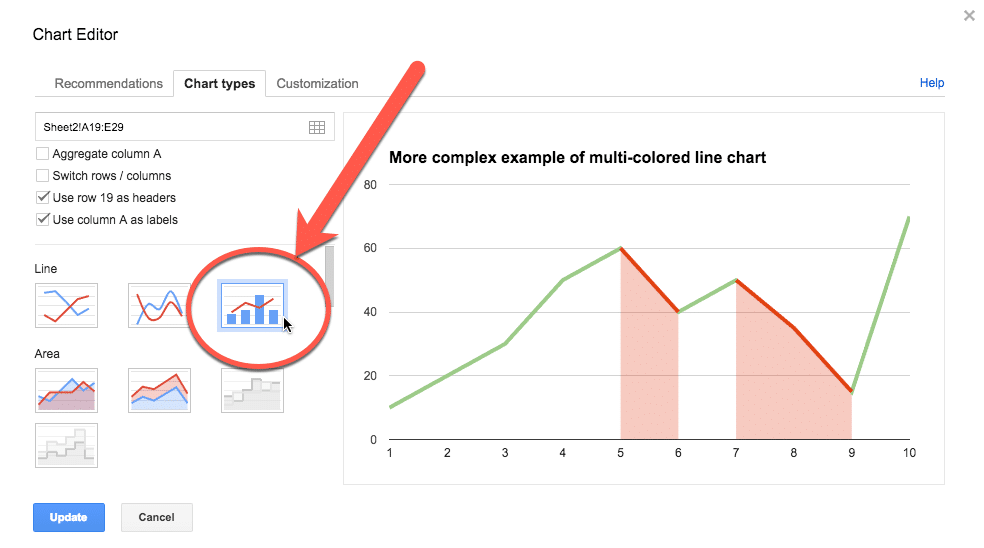Your final chart will look like this: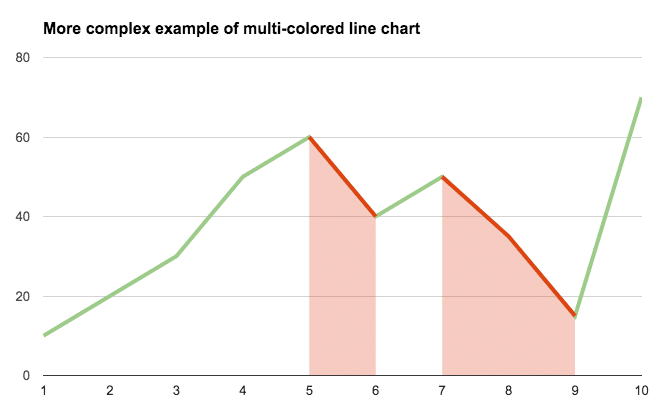And here’s the version with the inflection points marked: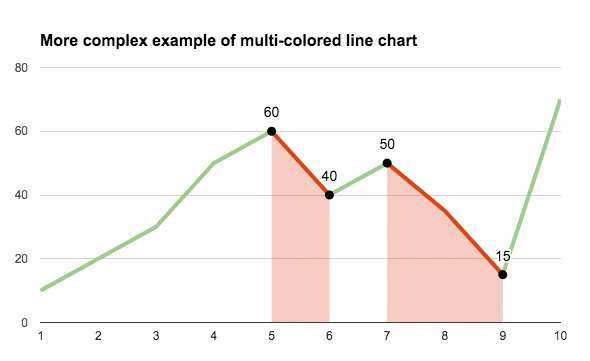## Can I see an example worksheet?

Yes, here you go.

## Reverse Text In Google Sheets

In this example, you’ll see how to reverse text in Google Sheets.

To start, enter some text into cell A1 and the formula in cell B1, to reverse the order of the text: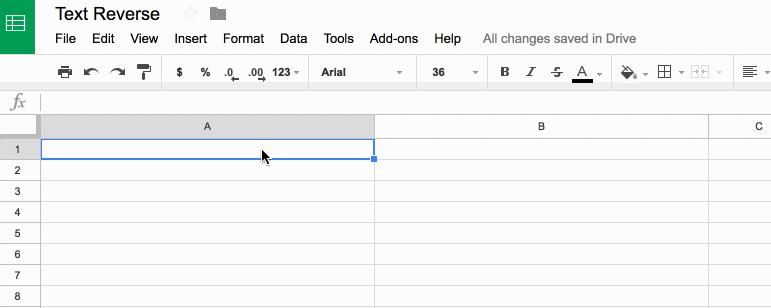## Reverse Text In Google Sheets

### What’s the formula?

`=ArrayFormula(IFERROR(PROPER(CONCATENATE(MID(A1,LEN(A1)-ROW(INDIRECT("1:"&LEN(A1)))+1,1))),""))`

It’s a beast! See below for a detailed breakdown of how it works.

It might be useful if you wanted to find the last character in a string, or the last occurrence of a character using the FIND() function.

Here’s an alternative, which reverses the capitalization too (submitted by Michael D over email – thanks!):

`=JOIN("",ARRAYFORMULA(MID(A1,LEN(A1)-ROW(INDIRECT("1:"&LEN(A1)))+1,1)))`

### How does this formula work?

Basically we make an array of numbers corresponding to how many letters are in the original text string. Then we reverse that, so the array shows the largest number first. Then we extract each letter at that position (so the largest number will extract the last letter, the second largest will extract the second-to-last letter, etc., all the way to the smallest number extracting the first letter). Then we concatenate these individual letters.

Easy! Err…

The only way to really understand this formula is to break it down, starting from the inner functions and building back out.

Assuming we have the text string “Abc” in cell A1, then let’s build the formula up in cell B1, step-by-step:

Step 1:

Use the LEN function to calculate the length of the text string and turn it into a range string with “1:”&LEN(A1)

Use the INDIRECT function to turn this string range reference into a valid range reference.

Finally wrap with ROW to convert into a row number list.

`=ROW(INDIRECT("1:"&LEN(A1)))`

which outputs a result of 1 in cell B1.

Step 2:

Turn the formula into an array formula, by hitting Ctrl + Shift + Enter, or Cmd + Shift + Enter (on a Mac), to the formula above. This will add the `ArrayFormula` wrapper around the formula:

`=ArrayFormula(ROW(INDIRECT("1:"&LEN(A1))))`

This outputs 1 in cell B1, 2 in cell B2 and 3 in cell B3: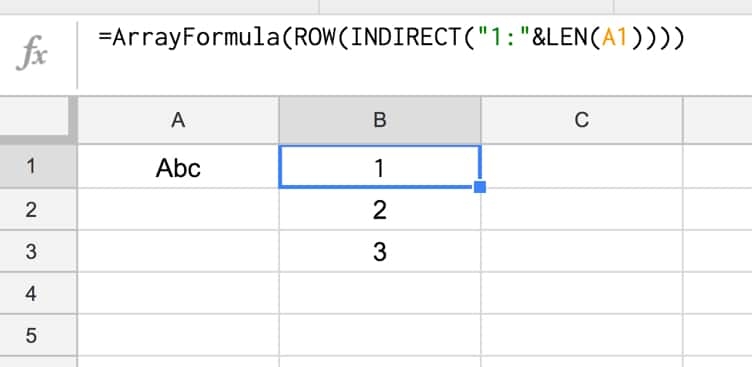Step 3:

Reverse the output, so 3 is in cell B1, 2 in B2 and 1 in B3, by subtracting from the length of the text in A1 and adding 1 to avoid 0:

`=ArrayFormula(LEN(A1)-ROW(INDIRECT("1:"&LEN(A1))) + 1)`

Step 4:

Use the MID formula to now extract the letters at position 3 (“c”), position 2 (“b”) and position 1 (“A”) and display in cells B1:B3:

`=ArrayFormula(MID(A1,LEN(A1)-ROW(INDIRECT("1:"&LEN(A1))) + 1 , 1))`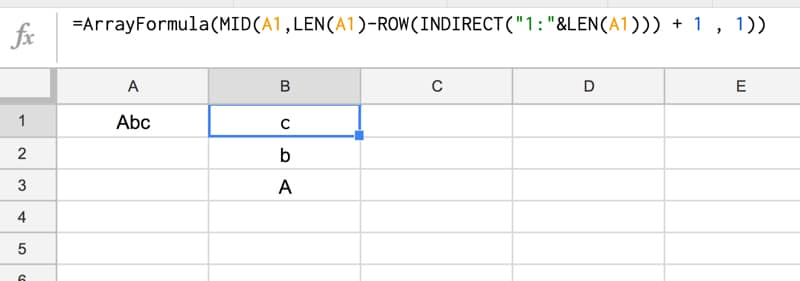Step 5:

Concatenate so that all the individual outputs are combined into a single cell:

`=ArrayFormula(CONCATENATE(MID(A1,LEN(A1)-ROW(INDIRECT("1:"&LEN(A1))) + 1 , 1)))`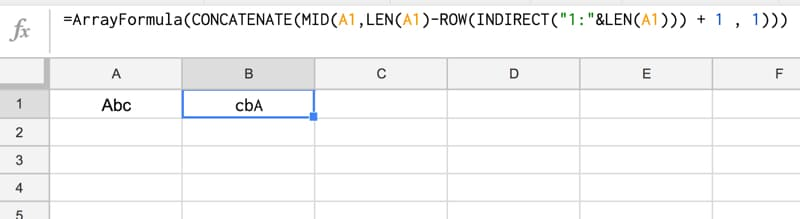Step 6 (optional):

The string is essentially reversed now, so we could stop here.

However, you can use the PROPER function to capitalize the first letters of each word only, for a true reverse effect:

`=ArrayFormula(PROPER(CONCATENATE(MID(A1,LEN(A1)-ROW(INDIRECT("1:"&LEN(A1))) + 1 , 1))))`

Step 7 (optional):

Last step is to add in an IFERROR function to avoid an unsightly error message if the input cell (A1) is blank:

`=ArrayFormula(IFERROR(PROPER(CONCATENATE(MID(A1,LEN(A1)-ROW(INDIRECT("1:"&LEN(A1)))+1,1))),""))`

Final output:

Here’s the formula in action again: